# Solving Functions for y

 When you are trying to graph a function such as 3x+y=10, it is easier if the function is solved for y. In this lesson, we will discuss how to do that. To solve a function for y, you use the same steps that you do for a normal equation. The only difference is that you have another variable that may need to be moved. Remember, to move something added, add the opposite to both sides. To move something multiplied or divided, do the inverse to both sides. Let's work with our example: 3x+y=10. Solving for y means that we have to get y by itself. Therefore, we have to move everything else to the other side. So let's first identify what needs to move. The 3x is on the same side as the y, so it needs to be moved. The 3x is being added to y. (Note that it does not matter that the 3 and x are being multiplied. All that matters is the relationship to y which is addition.) Since the 3x is being added, we will add the opposite to both sides: -3x. 3x-3x+y=10-3x On the left, 3x-3x=0, so they disappear. On the right, 10 and -3x are not like terms, so we cannot combine them, and we are almost finished. We just need torearrange the right hand side so thatit is in standard form. (This format is known as slope-intercept form.) y=10-3x y=-3x+10 Let's do a couple more examples: 1) 2y=3x-8In this case, the 2 is being multiplied by the y, so we will move it by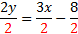doing the inverse: division. Please note that when you multiply or divide, you must distribute this to every term on both sides.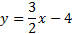Next, we'll simplify each term. The y is by itself, and it is in the correct form, so we are finished. 2) 5x+3y=12In this problem, we must move both the 5x and the 3. As before, we 5x-5x+3y=12-5xshould move what's being added first, so we will move the 5x 3y=12-5xby adding -5x to both sides.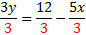Next, we must move the 3 by dividing every term by 3.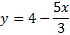Simplify and put in the correct form, and we are finished.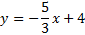(Notice that the negative stayed with the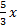) Practice:Solve each function for y. 1) y-4x=8 2) 3y=6x-12 3) -2y=3x-10 4) 3x+5y=10 5) -6x-y=2 Answers: 1) y=4x+82) y=2x-43)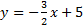4)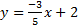5) -6x-2

 Related Links: Math Algebra Factors